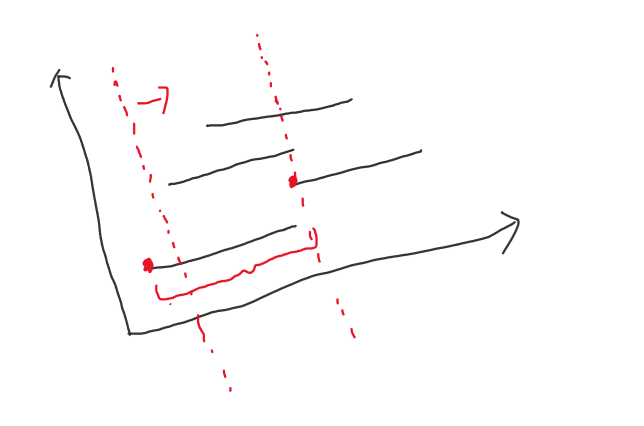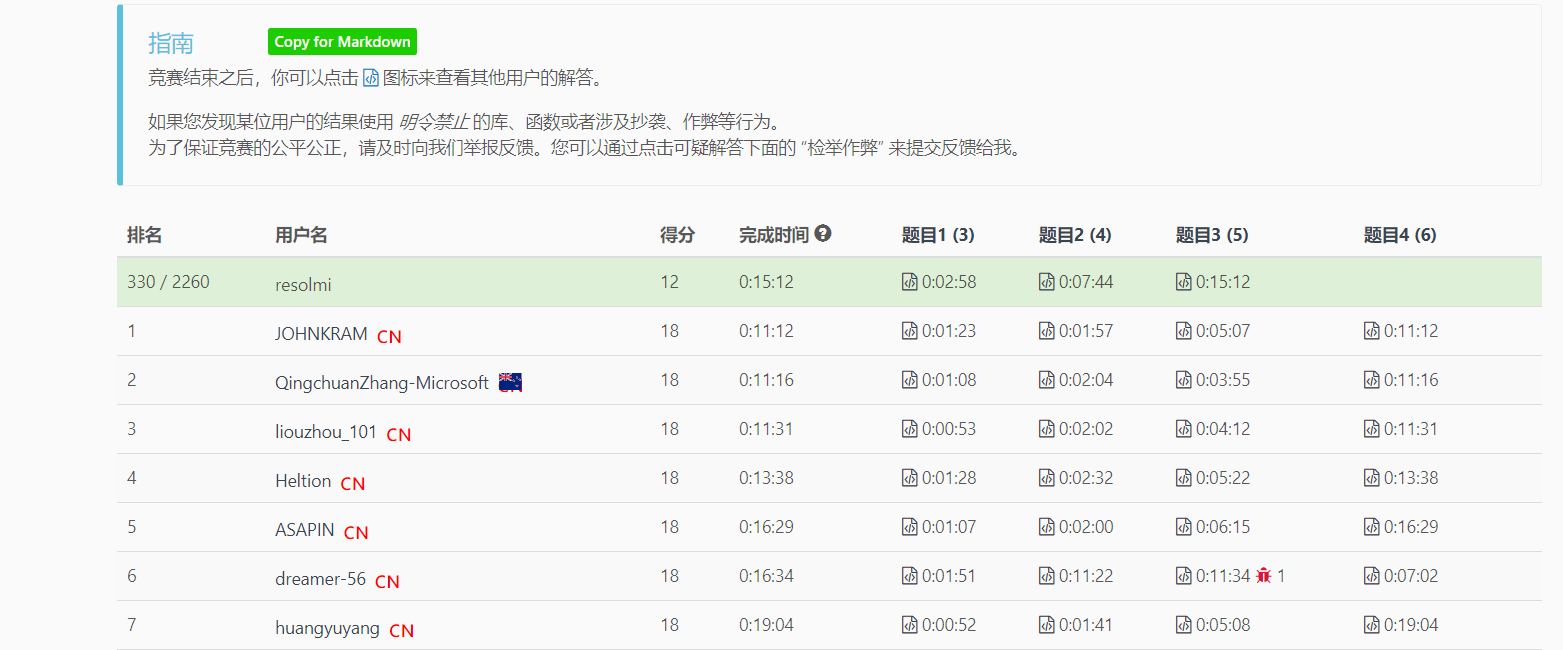## 239. 滑动窗口最大值

2020.2.9 回头看了下，这不是单调队列么😂（用堆也可以啦，不过既然要在头尾都操作，直接用队列会更方便）

[2，1，-1，3，……] k=3 ，当读到3这个元素的时候，窗口再向右移动最大值肯定不会是右边的元素了，所以直接剔除他们，在纸上画一画就明白了

i-list.getFirst()==k，i代表当前元素下标，上面的例子3对应的i就是3list.getFirst()=0，刚好差为k，就代表2已经超出窗口了应该移除了。

2ms，99% 提交记录这种做法是最快的😂，时间复杂度最坏应该是O(NK)

## 5312. 大小为 K 且平均值大于等于阈值的子数组数目

• 1 <= arr.length <= 10^5
• 1 <= arr[i] <= 10^4
• 1 <= k <= arr.length
• 0 <= threshold <= 10^4

19双周赛的第2题，很简单的滑动窗口，枚举所有窗口计数就行了

## 3. 无重复字符的最长子串20ms左右80%左右，思路也比较清晰，遍历字符串，然后从后往前遍历字符串，判断当前字符串在前面有没有，有的话就将前面相等那个元素(index)前的元素都移除（窗口右移）

eg: [head...index....tail] i 向右移动....index [head ... i]19/9/14，在网页上又写了一种不同的解法，属于解法4的变体（做的时候并没有想到解法4），freq[] 数组索引是字符串，但是值是该字符在s中对应的索引，不会遍历两遍数组，left可以通过索引直接跳到上一次出现的位置

map记录元素最后出现的位置，当重复的时候更新起点，只用遍历一遍

## 219. 存在重复元素 II

12ms，90%，其实效率的差距就在查找子串里有没有这个字符上，HashMap的containsKey的效率比我们遍历的不知道高到那里去了，底层源码暂时还看不太懂，以后看的时候再专门来讲

19.9.14又做了一遍，直接在网页上写的，本来应该是bugfree的，结果减反了。。。

19.9.15又做了一遍，这次代码写的很简洁，思路也不同了，直接利用set集合，维护一个大小为k的连续set(窗口)

## 209. 长度最小的子数组

2ms ，99%beats，一对比差距就出来了，上面这种做法就是利用了滑动窗口的思想，很巧妙的利用了上一次计算的值，不用重复的计算累加和，上面的那种方法每次都会重新计算累加和，但是很多都是重复的计算，所以浪费了很多时间，要注意边界条件

2 3 1 2 4 3 s=7Update(2020.5.4)

## 面试题57 - II. 和为s的连续正数序列

• 1 <= target <= 10^5

## 480. 滑动窗口中位数

47ms，70%左右，删除的时候利用二分删除，如果直接根据元素去删就跟数组效率差不多了。

## 76. 最小覆盖子串

• 如果 S 中不存这样的子串，则返回空字符串 “”。
• 如果 S 中存在这样的子串，我们保证它是唯一的答案。

10ms 86%，Hard题，其实大致的思路还是有的，主要是不知道怎么去和目标串对比，没想到用一个window数组去对比，一致想的是在目标串的数组上做手脚，但是越想越复杂。。。太蠢了😅，这题其实也可以用一个HashMap来做，但是我看了下提交记录上的普遍都是7,80ms，相对都比较慢，实际上题目没有明确的说明有特殊字符的话都是可以用一个ASCII数组来充当HashMap的，当然我这里用数组的时候相比HashMap要多了一步，需要统计不同字符出现的次数，不过这个操作也是常数级别的操作，并不耗时，整体时间复杂度O(N+M)，NM分别代表目标子串t 和源字符串 p的长度，首先遍历了t 然后滑动窗口，后面的滑动窗口左右边界最多移动2M次

Update

2020.4.15，在瞄了一眼之前做的之后按照之前的思路重写了一遍，感觉还行，有一个地方WA了一次

## 632. 最小区间

Difficulty: 困难

1. 给定的列表可能包含重复元素，所以在这里升序表示 >= 。
2. 1 <= k <= 3500
3. -105 <= 元素的值 <= 105## 438. 找到字符串中所有字母异位词

• 字母异位词指字母相同，但排列不同的字符串。
• 不考虑答案输出的顺序。

UPDATE:2020.7.23

15ms，86%时间复杂度O(M+N)，其实是完全套的之前最小覆盖子串的模板，不如肯定不好写这么长

## 567. 字符串的排列

1. 输入的字符串只包含小写字母
2. 两个字符串的长度都在 [1, 10,000] 之间

## 面试题 17.18. 最短超串

• big.length <= 100000
• 1 <= small.length <= 100000

## 1004. 最大连续1的个数 III

1. 1 <= A.length <= 20000
2. 0 <= K <= A.length
3. A[i]01

## 5434. 删掉一个元素以后全为 1 的最长子数组

Difficulty: 中等

• 1 <= nums.length <= 10^5
• nums[i] 要么是 0 要么是 1

29th双周赛的t3，秒切，其实是上一题1004. 最大连续1的个数 III的弱化## 424. 替换后的最长重复字符

UPDATE: (2020.5.5)

## 1234. 替换子串得到平衡字符串

• 1 <= s.length <= 10^5
• s.length 是 4 的倍数
• s 中只含有 ‘Q’, ‘W’, ‘E’, ‘R’ 四种字符

(update: 2020.4.15)

UPDATE: (2020.5.4)

## 1358. 包含所有三种字符的子字符串数目

• 3 <= s.length <= 5 x 10^4
• s 只包含字符 a，b 和 c 。

20双周赛T3

2020.5.4 用自己总结的滑窗模板重写，也是for-while结构，right和left不能同时扩展。比如"aaacb"这样的case

## 1423. 可获得的最大点数

• 1 <= cardPoints.length <= 10^5
• 1 <= cardPoints[i] <= 10^4
• 1 <= k <= cardPoints.length

186周赛T2，很明显的滑动窗口，前后拿K张最大，只需要求一个最小的[n-k]区间值就行了，也可以用前缀和，思路都一样

Tag里面有dp，确实这题和前面的石子游戏有一点像，但是还是滑窗来的比较直接。。

## 1208. 尽可能使字符串相等

s 中的第 i 个字符变到 t 中的第 i 个字符需要 |s[i] - t[i]| 的开销（开销可能为 0），也就是两个字符的 ASCII 码值的差的绝对值。

• 1 <= s.length, t.length <= 10^5
• 0 <= maxCost <= 10^6
• st 都只含小写英文字母。

## 1052. 爱生气的书店老板

• 1 <= X <= customers.length == grumpy.length <= 20000
• 0 <= customers[i] <= 1000
• 0 <= grumpy[i] <= 1

## 1040. 移动石子直到连续 II

1. 3 <= stones.length <= 10^4
2. 1 <= stones[i] <= 10^9
3. stones[i] 的值各不相同。

## 1456. 定长子串中元音的最大数目

• 1 <= s.length <= 10^5
• s 由小写英文字母组成
• 1 <= k <= s.length

## 1461. 检查一个字符串是否包含所有长度为 K 的二进制子串

Difficulty: 中等

• 1 <= s.length <= 5 * 10^5
• s 中只含 0 和 1 。
• 1 <= k <= 20

## 1498. 满足条件的子序列数目

Difficulty: 中等

• 1 <= nums.length <= 10^5
• 1 <= nums[i] <= 10^6
• 1 <= target <= 10^6

## 904. 水果成篮

Difficulty: 中等

1. 把这棵树上的水果放进你的篮子里。如果你做不到，就停下来。
2. 移动到当前树右侧的下一棵树。如果右边没有树，就停下来。

1. 1 <= tree.length <= 40000
2. 0 <= tree[i] < tree.length

## NC562.牛牛的魔法卡

• 1<=n<=10^6
• 1<=k<=50 0<=x<k
• 0 <= y <= 1e9

tag是二分，但是想了一会儿感觉好像没啥好的二分的思路，二分答案貌似可行，不过这题滑窗的思路更简单，类似76-最小覆盖子串滑就完事儿了

## 1375. 至少K个不同字符的子串（LintCode）

• 10 ≤ length(S) ≤ 1,000,000
• 1 ≤ k ≤ 26

## 1675. 数组的最小偏移量

Difficulty: 困难

• 如果元素是偶数 ，除以 2
• 例如，如果数组是 [1,2,3,4] ，那么你可以对最后一个元素执行此操作，使其变成 [1,2,3,2]
• 如果元素是奇数 ，乘上 2
• 例如，如果数组是 [1,2,3,4] ，那么你可以对第一个元素执行此操作，使其变成 [2,2,3,4]

• n == nums.length
• 2 <= n <= 105
• 1 <= nums[i] <= 109